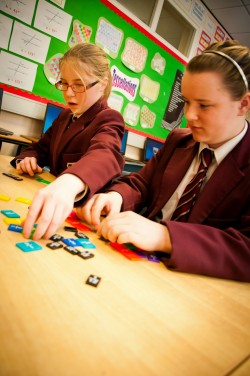# Maths

Hello, welcome to the Year 8 Maths page.

This page will give you some information about things that you will study during your first year in St Paul’s. Whether or not you have studied this subject at primary school, we’re sure you will find the information below helpful.In Mathmetics, we cover the following topics during Year 8:

• Numeracy: The Four Rules revisited
• Graphs: Conversion graphs
• Statistics: Diagrams / Pie charts / Questionnaires
• Estimating: Powers and roots / Menta maths / Estimation
• Algebra: Number patterns / Function machines
• Transformations: Reflections / Translations / Rotations / Enlargement
• Angles: Parallel lines / Polygons / Bearings
• Negative Numbers: Ordering / Rounding
• Percentages & Fractions: Calculating percentages / Relating percentages / fractions and decimals
• Probability: Number line / Probability spaces
• Ratio: Proportion / Maps and Scales
• Area: Perimeter and area
• Volume: Prisms
• Straight lines: Gradients of straight lines / Intersecting lines
• Statistics: Averages / Range / Frequency polygons
• Trial and improvement: Inequalities / Order on a number line